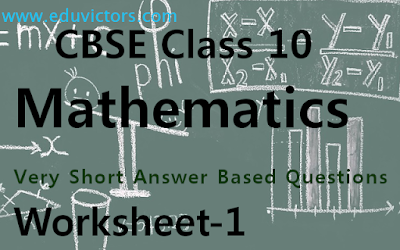## CBSE Class 10 - Maths - Very Short Answer Based Questions

(Solved Worksheet-1)

Q1: What is the maximum no. of factors of a prime number?

Q2: What is the L.C.M of x and y if y is a multiple of x?

Q3: Find the zeroes of the polynomial x²-1.

Q4: For what value of ‘k’ the pair of equations x-ky+4=0 and 2x-4y-8=0 is inconsistent?

Q5: Determine k, so that the equation x²-4x+k=0 has no real roots.

Q6: Which term of the sequence 4,9,14...is 124?

Q7: Find the sum of first 10 multiples of 5.

Q8: If θ = 45°, find the value of sec² θ.

Q9: The ordinate of point A on y-axis is 5 and B has co-ordinates (-3, 1). Find the length of AB.

Q10: Find the area of ΔABC where A (2,3), B (-2,1) and C (3,-2)

Q11: Are two triangles with equal corresponding sides always similar?

Q12: The perimeters of two similar triangles ABC and PQR are respectively 36 cm and 24 cm. If PQ = 8 cm, then the value of AB is ____.

Q13: How many tangents can be drawn to a circle from a point outside the circle?

Q14: If the angle between two radii of a circle is 130°, then what is the angle between the tangents at the end points of radii at their point of intersection?

Q15: A wire in the shape of square of perimeter 88 cm. is bent so as to form a circular ring. Find the radius of the ring.

Q16: To divide the line segment AB in the ratio 2:3, a ray AX is drawn such that ∠BAX is acute, AX is then marked at equal intervals. Find minimum number of these marks.

Q17: Write a sample space when two coins are tossed simultaneously?

Q18: What is the surface area of a cube whose volume is 64 cm³?

Q19: Give the empirical relation between median, mode and mean.

Q20:  If the median of a series exceeds the mean by 3, find by what number the mode exceeds its mean?

Answers:

Answer 1: 2 (self and 1)

Answer 2: y

Answer 3: +1, -1
x²-1 = 0
(x + 1)(x - 1) = 0
⇒ x = -1 or x = 1

Answer 4: k = 2

Answer 5: k > 4
D < 0 for no real roots
b² - 4ac < 0
b² - 4ac < 0
(-4)² - 4(1)(k) < 0
16 -4k < 0
4 - k < 0
k > 4

Answer 6: n = 25

Answer 7: S = 275

Answer 8: 2

Answer 9: 5 units
A(0, 5) and B(-3, 1)
AB = √(-3 - 0)² + (1 - 5)² =  √(9 + 16) = √25
AB = 5 units

Answer 10: 11 square units

Answer 11: No, Included angles should be equal.

Answer 12: 12 cm

Answer 13: Two

Answer 14:  50°
Angle at the point of intersection of tangents = 180° — 130° = 50°.

Answer 15: 14 cm

Answer 16: Minimum number of marks = 2 + 3 = 5

Answer 17: S = { HH,HT,TH,TT}

Answer 18: 16 cm²

Answer 19: Mode = 3 median – 2 Mean

Answer 20: Exceeds by 9

Given, Median = Mean + 3
We know that, Mode = 3 Median -2Mean
= 3 (Mean + 3)-2Mean
⇒ Mode = Mean +9

Hence Mode exceeds Mean by 9.

☞See also:#### Post a comment

We love to hear your thoughts about this post!